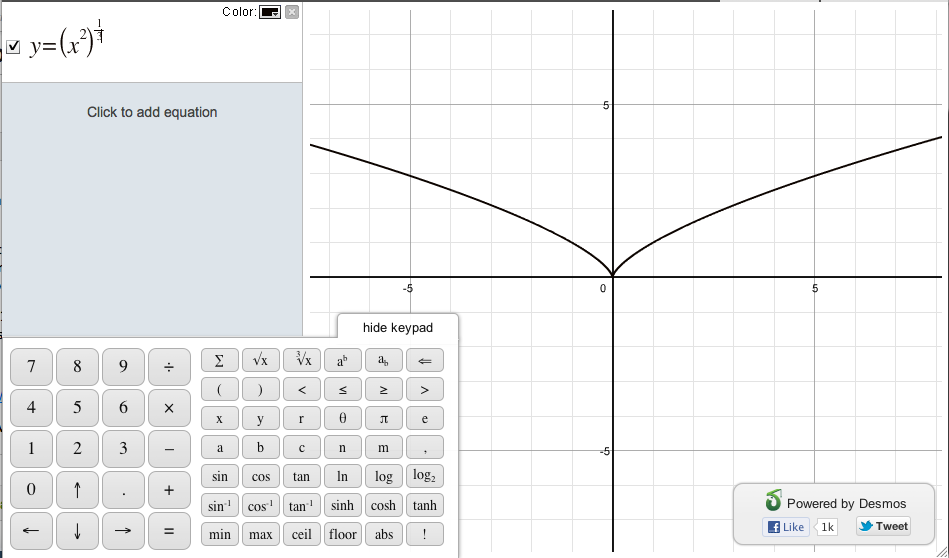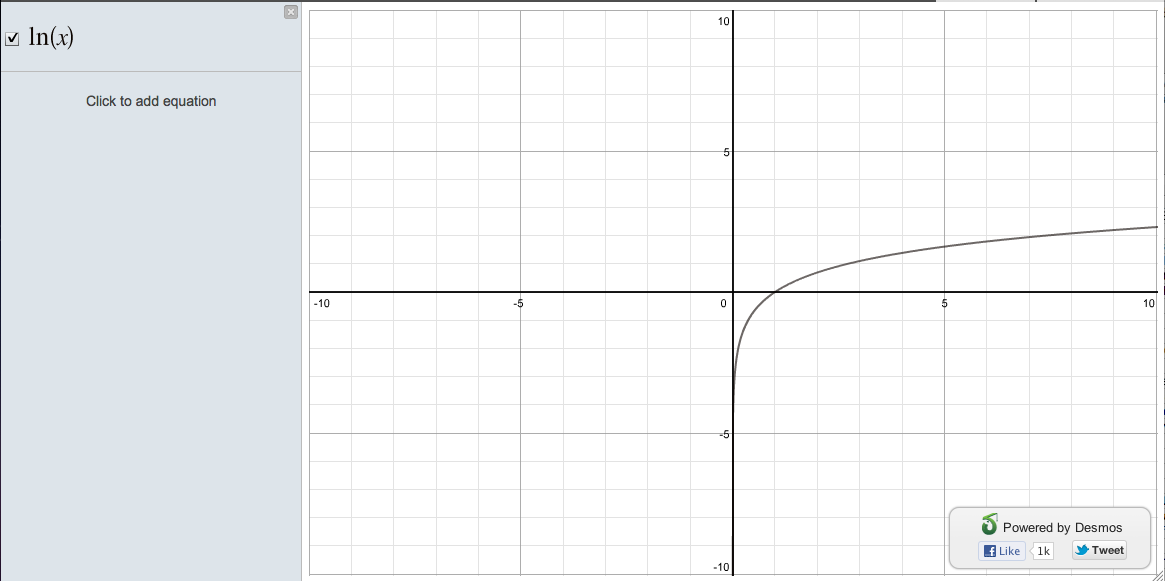# Graphing Fractional Powers

We get a great question in on twitter today from @MathG8 regarding this graph:The question posed was:

Find the Max and Min values of f(x)=x^(2/3) on the interval [-1,8] by considering the graph of f.

And the trouble? We only show from [0, 8]! Why do we do that?

====

[Warning: this part is going to get technical]:

Oh, the arguments we had about this graph (this is what we argue about in our office). Here’s the trouble. Everyone knows that sqrt(x) doesn’t give a real answer for negative numbers [does it?]. But what about x^(2/3)? Well, it depends! Here are some different ways to write this expression:

(x^2)^(1/3). In this case, you’re taking the cube root of a positive number. Easy! It looks like this:But how about (x^(1/6))^4? That looks like the same fraction (in different terms), but the 6th root of (-1) has all sorts of different possible answers (well, 6 of them), all of which are imaginary! What to do then?

Let’s take a step back. Calculating integer powers is easy: x^2 = xx. x^3 = xxx. But how do we actually calculate x^(2/3)? One way, which is what calculators tend to use (ours included!), is to use logarithms. Say:

y = x^(2/3). Log of both sides yields:

log(y) = log(x^(2/3)) or, using our power rules, ln(y) = (2/3)ln(x). Exponentiating both sides, yields: y = exp[(2/3) ln(x)], which a calculator can easily find. That is, if “x” is positive. Otherwise, what is ln(x)?

And here is where Wolfram Alpha and we diverge. Here’s our graph of ln(x):And Wolfram Alpha’s:What’s going on here? Wolfram alpha is showing a partially imaginary answer for log(-1). While this is technically correct as one of an infinite number of correct answers to log(-1), this is not what most people expect when they take the log of “-1”. There is no real (non-imaginary) answer to that question. So we’ve decided to return “Not a Number.”

There’s an advantage to Wolfram Alpha’s method: they can return an answer for (-1)^(2/3)! So, let’s take a look at WolframAlpha’s graph of x^(2/3):Oh boy. They are returning an answer, but it sure isn’t the one you expect. They’re saying that (-1)^(2/3) = -.5 + isqrt(3)/2. Yikes. They could just as well have chosen to say that (-1)^(2/3) = 1, but really those two answers are equally correct. The trouble is, once you allow imaginary answers, the correct* answer becomes ambiguous. Our solution: we just don’t show fractional roots of negative numbers. We actually hard-coded in the “cube root” function (cbrt(x)) which does show both sides. Perhaps we should do that for some fractional powers too (like this one)? Let us know what you think on our facebook page: http://www.facebook.com/desmosinc

====

&tldr; evaluating y = x^(2/3) on [0,8] is pretty straightforward. But once you get into the negatives, you’re opening quite the can of worms…

Thanks @MathG8! And always feel free to tweet us more questions or mathematical quirks @desmosinc

- Eli & Team Desmos